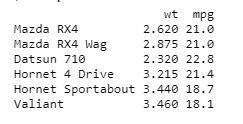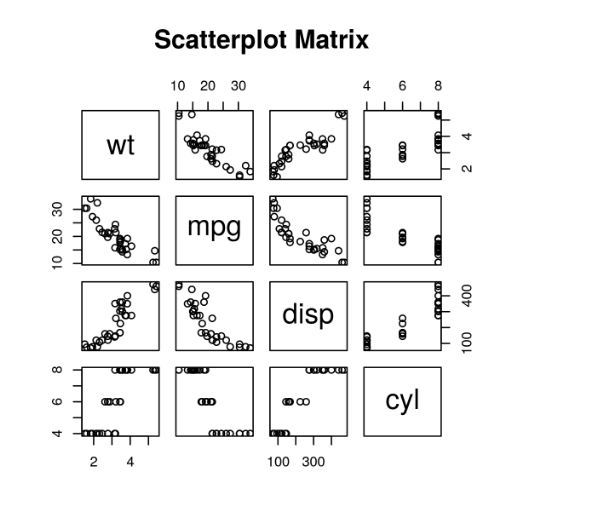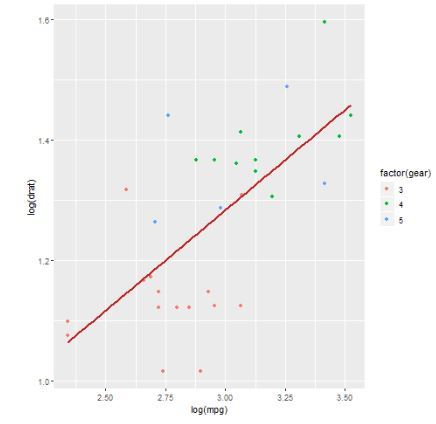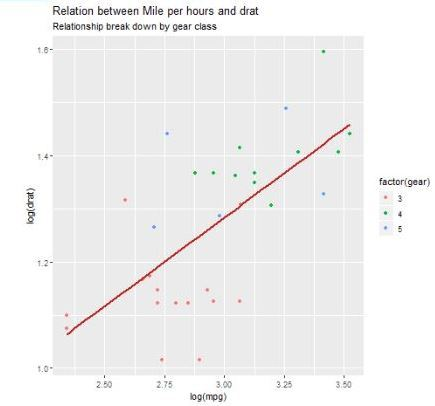# Scatter plots in R Language

• Last Updated : 21 Apr, 2020

A scatter plot is a set of dotted points to represent individual pieces of data in the horizontal and vertical axis. A graph in which the values of two variables are plotted along X-axis and Y-axis, the pattern of the resulting points reveals a correlation between them.

The simple scatterplot is created using the plot() function.

Syntax:

`plot(x, y, main, xlab, ylab, xlim, ylim, axes)`

Parameters:

• x: This parameter sets the horizontal coordinates.
• y: This parameter sets the vertical coordinates.
• xlab: This parameter is the label for horizontal axis.
• ylab: This parameter is the label for vertical axis.
• main: This parameter main is the title of the chart.
• xlim: This parameter is used for plotting values of x.
• ylim: This parameter is used for plotting values of y.
• axes: This parameter indicates whether both axes should be drawn on the plot.

#### Simple Scatterplot Chart

Approach: In order to create Scatterplot Chart:

1. We use the data set “mtcars”.
2. Use the columns “wt” and “mpg” in mtcars.

Example:

 `input` `<``-` `mtcars[, c(``'wt'``, ``'mpg'``)]``print``(head(``input``))`

Output:#### Creating a Scatterplot Graph

Approach: In order to create Scatterplot graph:

1. We are using the required parameters to plot the graph.
2. In this ‘xlab’ describes the X-axis and ‘ylab’ describes the Y-axis.

Example:

 `# Get the input values.``input` `<``-` `mtcars[, c(``'wt'``, ``'mpg'``)]``  ` `# Plot the chart for cars with ``# weight between 1.5 to 4 and ``# mileage between 10 and 25.``plot(x ``=` `input``\$wt, y ``=` `input``\$mpg,``     ``xlab ``=` `"Weight"``,``     ``ylab ``=` `"Milage"``,``     ``xlim ``=` `c(``1.5``, ``4``),``     ``ylim ``=` `c(``10``, ``25``),         ``     ``main ``=` `"Weight vs Milage"``)``     `

Output:#### Scatterplot Matrices

When we have two or more variables and we want to correlate between one variable and others so we use a scatterplot matrix.

pairs() function is used to create matrices of scatterplots.

Syntax:

`pairs(formula, data)`

Parameters:

• formula: This parameter represents the series of variables used in pairs.
• data: This parameter represents the data set from which the variables will be taken.

Example:

 `# Plot the matrices between 4 variables giving 12 plots.`` ` `# One variable with 3 others and total 4 variables.``pairs(~wt ``+` `mpg ``+` `disp ``+` `cyl, data ``=` `mtcars,``       ``main ``=` `"Scatterplot Matrix"``)`

Output:#### Scatterplot with fitted values

Approach: In order to create Scatterplot Chart:

1. We are using the ggplot2 package provides ggplot() and geom_point() function for creating a scatterplot.
2. Also we are using the columns “wt” and “mpg” in mtcars.

Example:

 `# Loading ggplot2 package  ``library(ggplot2)  ``   ` `# Creating scatterplot with fitted values.  ``# An additional function stst_smooth ``# is used for linear regression.  ``ggplot(mtcars, aes(x ``=` `log(mpg), y ``=` `log(drat))) ``+` `           ``geom_point(aes(color ``=` `factor(gear))) ``+` `           ``stat_smooth(method ``=` `"lm"``,``           ``col ``=` `"#C42126"``, se ``=` `FALSE, size ``=` `1``)  `

Output:#### Adding title with dynamic name

Approach: To create Scatterplot Chart, Adding a sub-title:

1. We use additional function, In ggplot we add the data set “mtcars” with this adding ‘aes’, ‘geom_point’.
2. Use the Title, Caption, Subtitle.

Example:

 `# Loading ggplot2 package  ``library(ggplot2)  ``   ` `# Creating scatterplot with fitted values.  ``# An additional function stst_smooth ``# is used for linear regression.  ``new_graph<``-``ggplot(mtcars, aes(x ``=` `log(mpg), y ``=` `log(drat))) ``+``                      ``geom_point(aes(color ``=` `factor(gear))) ``+` `                      ``stat_smooth(method ``=` `"lm"``, col ``=` `"#C42126"``, ``                      ``se ``=` `FALSE, size ``=` `1``)  `` ` `# in above example lm is used for linear regression ``# and se stands for standard error.  ``# Adding title with dynamic name  ``new_graph ``+` `labs(  ``        ``title ``=` `"Relation between Mile per hours and drat"``,  ``        ``subtitle ``=` `"Relationship break down by gear class"``,  ``        ``caption ``=` `"Authors own computation"` `) `

Output:My Personal Notes arrow_drop_up